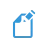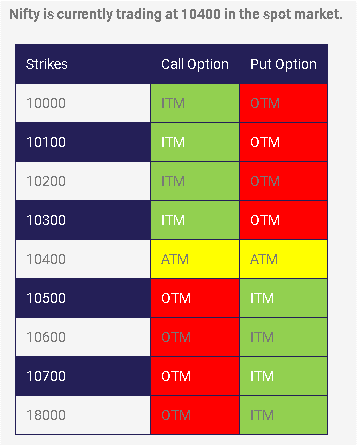# Option Moneyness - ITM, OTM and ATM

Option Moneyness is an indicator that tells us whether an option will make money for us if we exercise it at present.

On the basis of moneyness, options can be categorized into three categories:

• ITM (In the money)
• ATM (At the money)
• OTM (Out of the money)

To understand the concepts of ITM, OTM, and ATM, we first need to understand the concepts of strike price in options, spot price, and intrinsic value.Table of Contents
• What is Intrinsic Value of an Option?
• Significance of Intrinsic Value

## What is Intrinsic Value of an Option?

To understand the concept of Intrinsic Value, we first need to understand what we mean by strike price and spot price.

• Strike Price: It is the price at which the buyer and seller of an option contract have decided to trade the underlying security at a future date. It is fixed in the contract and does not fluctuate. The strike prices are decided by the exchange.
• Spot Price: It is the price at which the underlying security is trading at the present moment (i.e. when option buyer decides to execute the contract).

Now, Intrinsic Value of an option is the money that a buyer can make from an option if he executes it right then (if he has the right to exercise that option on that particular day). Intrinsic Value of an option is always positive. That is:

Intrinsic Value = The difference between current spot price and strike price of the underlying security

To be more precise:

• Call option Intrinsic Value: Current Spot Price – Strike Price
• Put option Intrinsic Value: Strike Price – Current Spot Price

Higher the intrinsic value, deeper the moneyness of the option.

The profit/loss that a trader makes upon sale (or exercise) of an option = Intrinsic Value – Premium

So, to gain profit in options trading, we need to consider both the strike price and the premium to be paid by us. Keep both in mind when you buy an option, be it call or put.

### ITM (In the money options)

• A call option is said to be in ITM if: Strike price of the security < Current spot price of the security.
• A put option is said to be ITM if: Strike price of the security > Current spot price of the security.

### ATM (At the money options)

• A call option is said to be in ATM if: Strike price of the security = Current spot price of the security (or nearby).
• A put option is said to be ATM if: Strike price of the security = Current spot price of the security (or nearby).

### OTM (Out of the money options)

• A call option is said to be in OTM if: Strike price of the security > Current spot price of the security.
• A put option is said to be OTM if: Strike price of the security < Current spot price of the security.

As Intrinsic value cannot be negative, intrinsic value of OTM options is considered zero. This is because option buyers cannot book more losses than the premium paid by them. It will become more clear with some examples later on in this article.We can summarize it as follows:

• The strike that is closest to the current spot price is called ‘At the money’. So, there can only be one ATM strike. Its intrinsic value will obviously be the smallest (it may be positive, or even zero if strike price = spot price).
• If the intrinsic value is a positive number, then the option strike is considered ‘In the money’. In case of call options, all option strikes that are below the ATM strike are considered ITM (as being below the ATM means that they are going to be below the spot price too). In case of put options, all option strikes that are higher than the ATM strike are considered ITM (as being above the ATM means that they are going to be above the spot price too).
• If the intrinsic value = 0, then the option strike is considered ‘Out of the money’. In case of call options, all option strikes that are higher than the ATM strike are considered OTM (as being above the ATM means that they are going to be above the spot price too). In case of put options, all option strikes that are lower than the ATM strike are considered OTM (as being below the ATM means that they are going to be below the spot price too).Note

Some experts also classify Moneyness of an option into five categories:

• Deep In the money
• In the Money (ITM)
• At the Money (ATM)
• Out of the Money (OTM)
• Deep Out of the Money

As we traverse from ‘Deep ITM’ option to ‘Deep OTM’ option, the premium keeps on decreasing. In other words, we need to pay more premiums for ITM options than for OTM options, as ITM options have positive intrinsic value.

If we assume that the price moves in the favourable direction, the ITM options will get into the profit zone before the ATM option. The OTM options will get into the profit zone at the last. That’s why OTM options have the cheapest premiums.

However, many experts consider it the best to trade in ATM. That’s because, the premium to be paid is comparatively lower than ITM options, and if the price moves in the favourable direction, you will still make huge profits.

### Examples

If Nifty 50 is trading at the spot price of 16415 CE and the strike price is 16400 CE, the premium paid is Rs. 5, and you have the right to exercise this option today. Then:

The call option is in ITM as: Strike price of the security < Current spot price of the security.
Call option Intrinsic Value = Current Spot Price – Strike Price = 16415 - 16400 = Rs. 15
Profit = Intrinsic Value – Premium = 15 – 5 = Rs. 10

Let’s consider another example. If Nifty 50 is trading at the spot price of 16385 CE and the strike price is 16400 CE, the premium paid is Rs. 5, and you have the right to exercise this option today. Then:

The call option is in OTM as: Strike price of the security > Current spot price of the security.
Call option Intrinsic Value = Current Spot Price – Strike Price = 16385 - 16400 = - Rs. 15
But as intrinsic value cannot be negative, we will consider it to be zero.
Loss = Intrinsic Value – Premium = 0 – 5 = - Rs. 5 (loss will be equal to the premium paid; it cannot be higher than the paid premium)

Now, let’s have a look at put options.

If Nifty 50 is trading at the spot price of 16380 PE and the strike price is 16400 PE, the premium paid is Rs. 5, and you have the right to exercise this option today. Then:

The put option is in ITM as: Strike price of the security > Current spot price of the security.
Put option Intrinsic Value = Strike Price – Current Spot Price = 16400 - 16380 = Rs. 20
Profit = Intrinsic Value – Premium = 20 – 5 = Rs. 15

If Nifty 50 is trading at the spot price of 16420 PE and the strike price is 16400 PE, the premium paid is Rs. 5, and you have the right to exercise this option today. Then:

The put option is in OTM as: Strike price of the security < Current spot price of the security.
Put option Intrinsic Value = Strike Price – Current Spot Price = 16400 - 16420 = - Rs. 20
But as intrinsic value cannot be negative, we will consider it to be zero.
Loss = Intrinsic Value – Premium = 0 – 5 = - Rs. 5 (loss will be equal to the premium paid; it cannot be higher than the paid premium)Note

We buy options in lots. So, all of these values (intrinsic value, premium, profit/loss) have to be multiplied by the size of the lot to find out our actual profit/loss.Option Chain

In most of the exchanges and trading platforms, you will see a list of all the available strikes for a particular underlying asset. They would often be classified based on their moneyness. Here you will also get to see some essential extra data for each one of these option strikes, such as:

• volumes
• open interest, etc.

## Significance of Intrinsic Value

We must know the intrinsic value (or moneyness) of an option before we buy it. The more the intrinsic value, the more are the chances that we will book profits. That’s why options having more intrinsic value have higher premiums too.

So, in a way, finding the intrinsic value of an option allows you to:

• decide which strike to trade.
• estimate whether the premium to be paid by you is justified or not.

Also, there are certain market forces, called Option Greeks, that act on option strike prices. Option Greeks affect the premium associated with option strikes. They affect ITM, ATM, and OTM option strikes in different ways. So, understanding the concept of moneyness of options also helps us to understand Option Greeks and their impacts on premiums. We have covered Option Greeks in a separate article of ours.

Share on: